# LDR sensor control with ArduinoHello friends, Welcome to my SriTu Hobby blog. So far we have described 20 articles through the blog. Each of these articles explains the basics of Arduino components. You can read these previous articles. So let’s go to today’s post. Today we are going to talk about the LDR sensor.

What is an LDR sensor?

It is called a Light Dependent Resistor. It is a resistor. This is also known as a photoresistor. This is a semiconductor device. Depending on the amount of light falling on the sensor surface, the resistance value increases or decreases. It depends on the electron value. The higher the light, the higher the electron value, and the lower the light, the lower the electron value. As the electron value increases, the resistance value decreases as the current is easier to flow. When the electron value decreases, the resistance value increases as the current becomes difficult to flow. We can use this method to create light-sensitive circuits. This sensor is also used for night-active street lamps. We can use this sensor for various projects. It depends on your creativity. Ok, let’s check this sensor activity using a multimeter. Use a multimeter and sensor for this.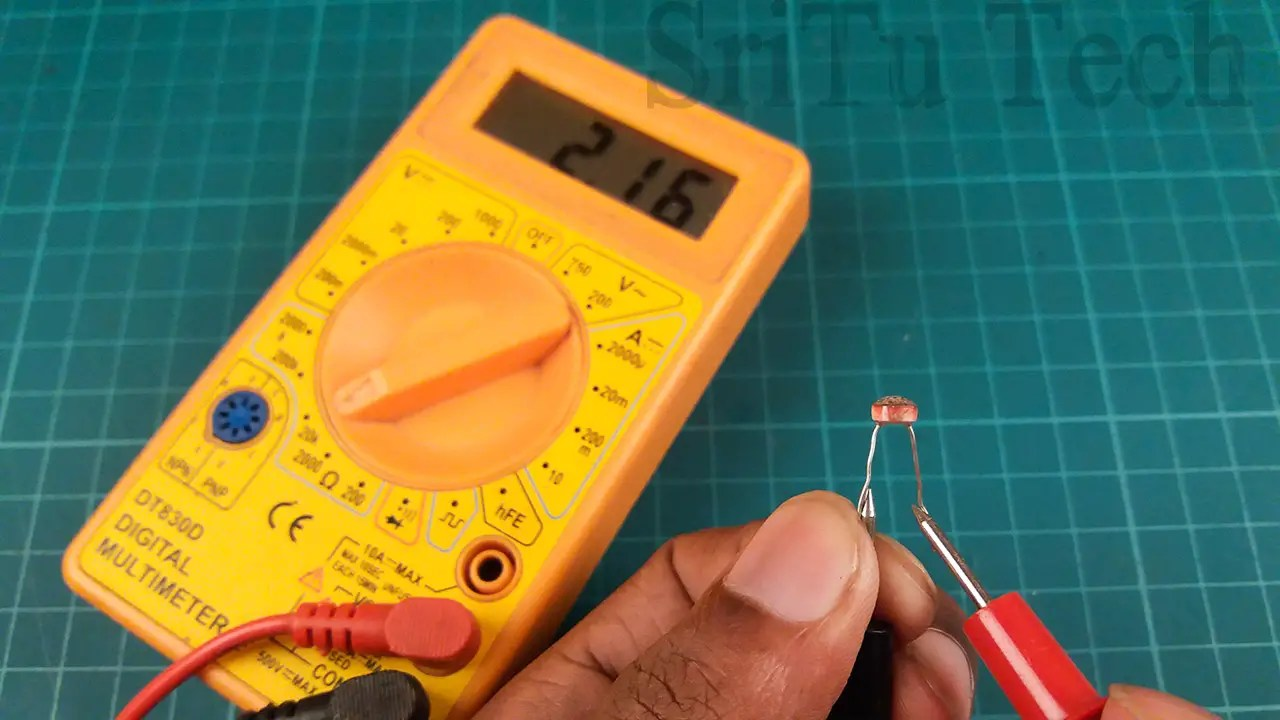First time rotate to multimeter 20k ohm scale. After, connect the sensor legs to the multimeter probes. Now you can see the sensor resistance value through the multimeter. Cover the sensor by hand and see how the value changes.
Ok, let’s look at now how to connect the Arduino board to this sensor. We have to use a special method to get the values ​​through this sensor. That is, we need to create a voltage divider circuit. It depends on the sensor resistance value. We need only a resistor for this circuit. When this is given a potential of 5 volts, the values ​​can be obtained from the voltage difference in the voltage divider point. For that, we can use analog pins on the Arduino board. Today let’s see how to blink a LED bulb using LDR sensor values. The required components are as follows.

### Components

Let’s do this project step by step.

### Step 1

Let us identify these components.

Arduino Uno board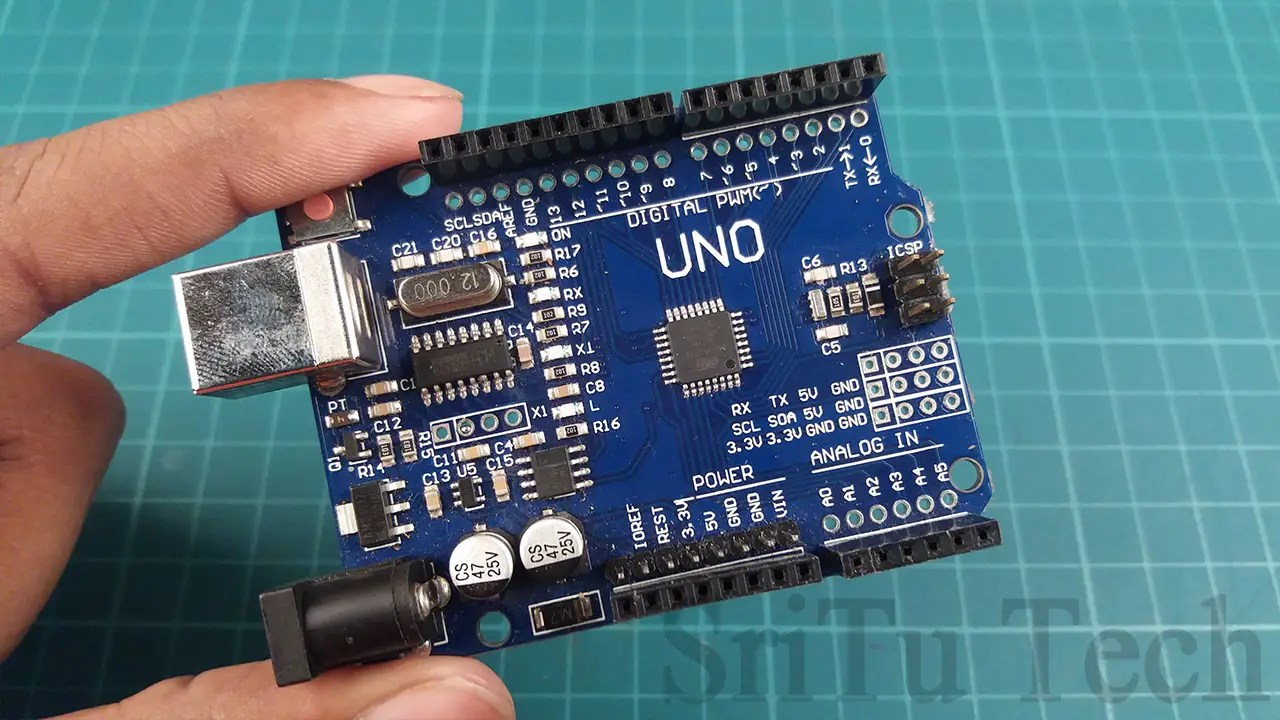You can use any other board for this project.

LDR sensorThis component is described above.
LED bulb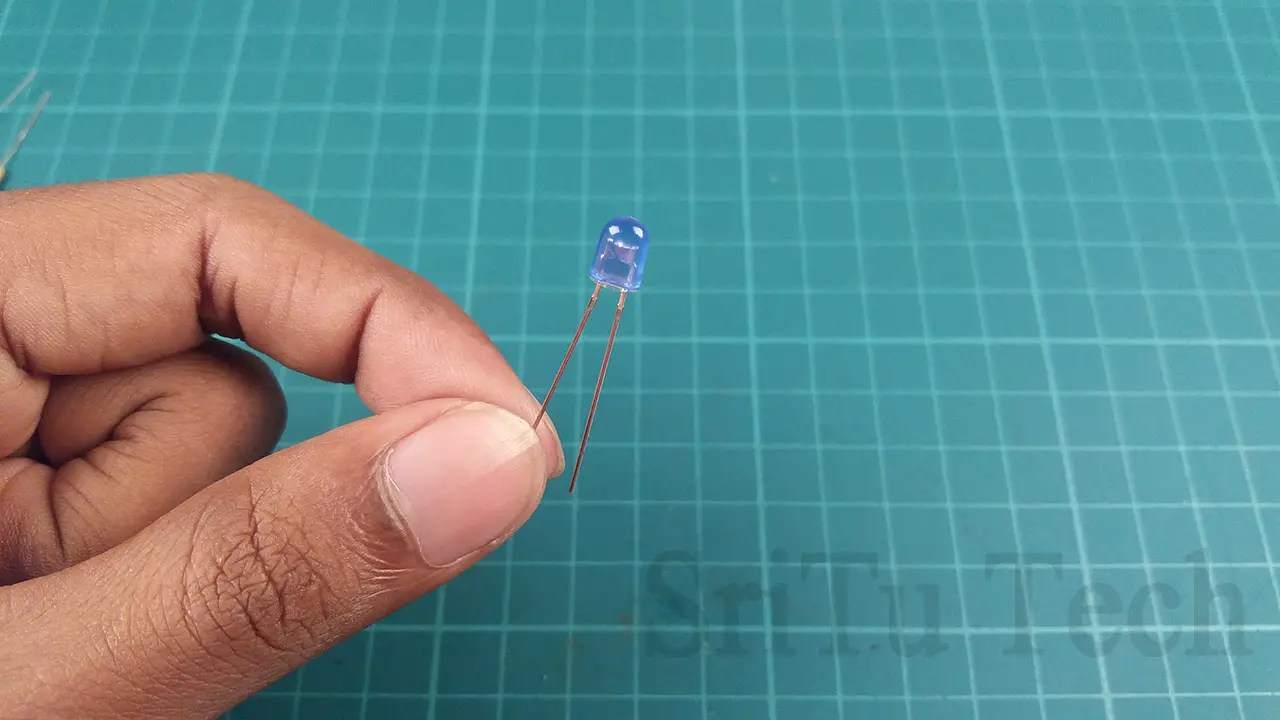Use to look after the outputs.

180ohm resistor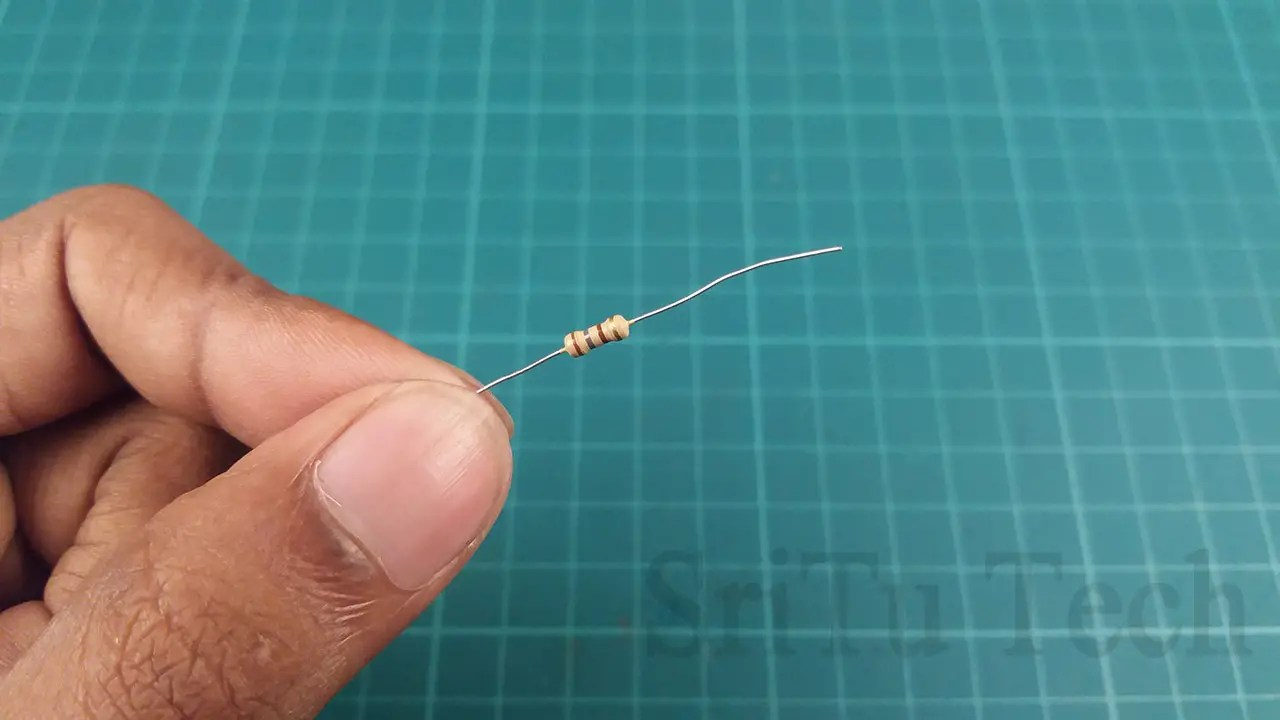Use this component to control the current in the LED bulb.

10k resistor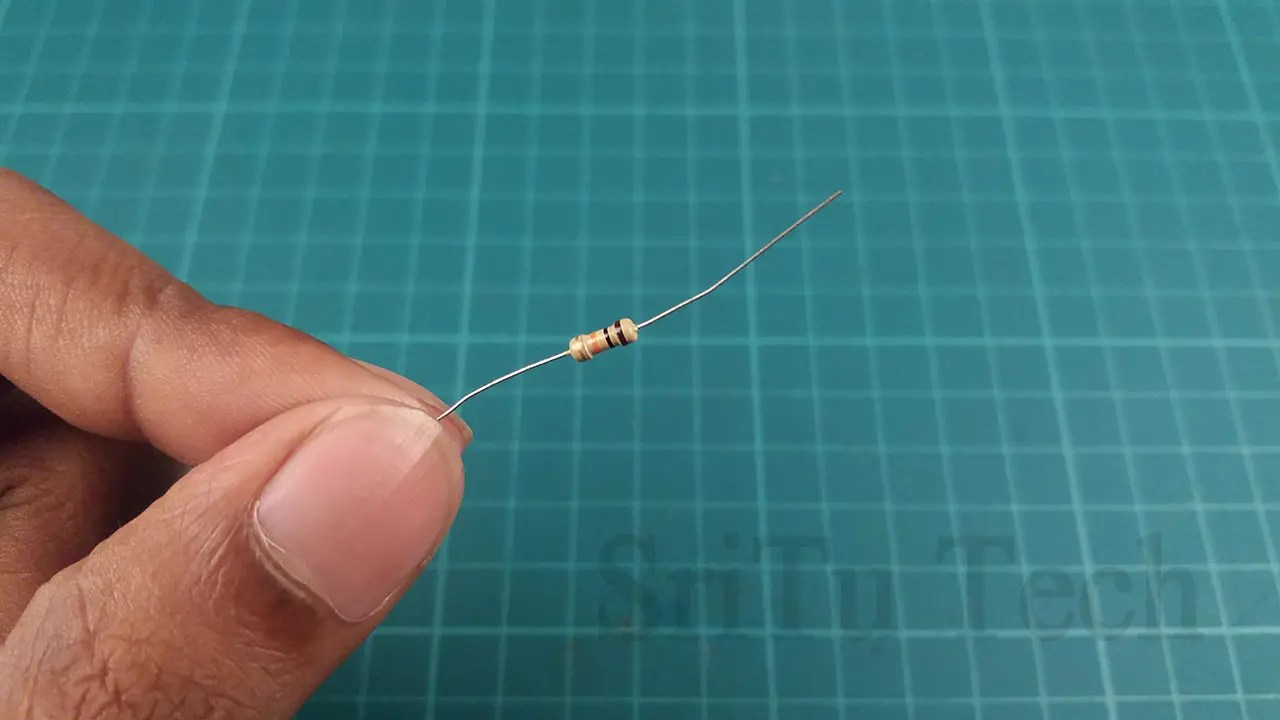Use this component in the voltage divider circuit.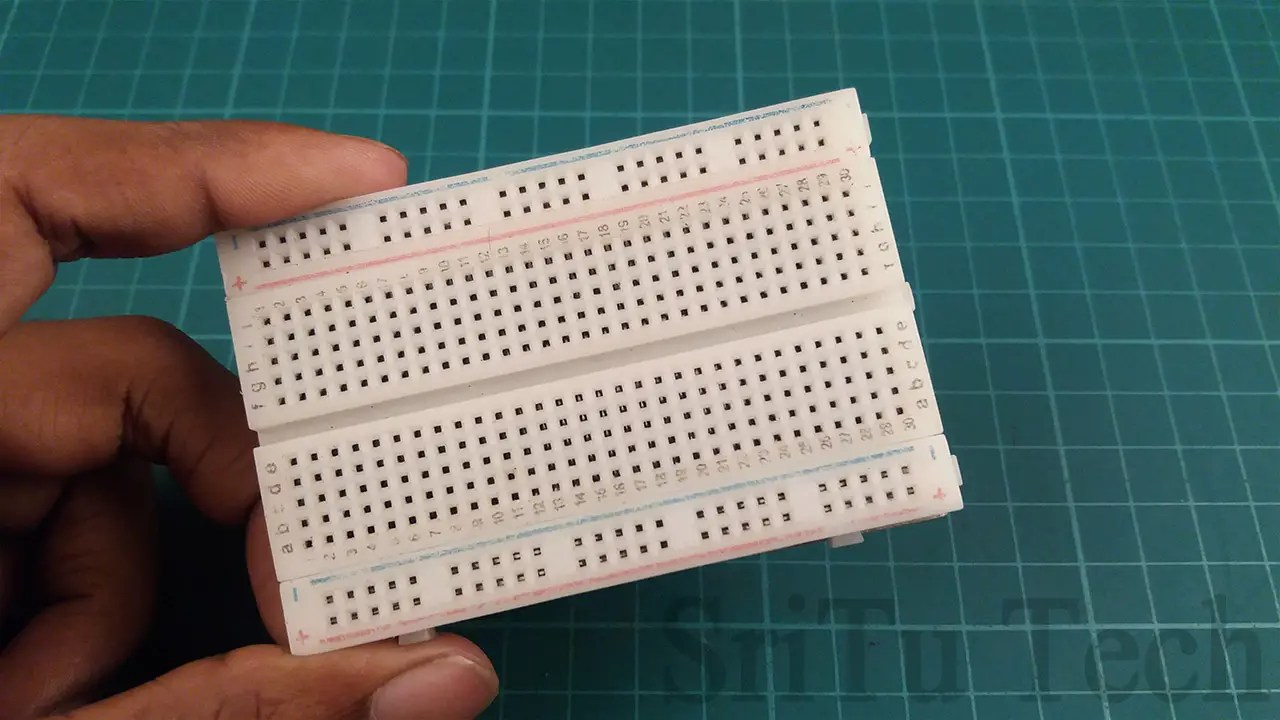Use this to connect components.

Jumper wiresYou can use five MALE to MALE jumper wires for this project.

### Step 2

Connect these components using the circuit diagram below. Remember to connect the LED bulb with the PWM pin. Use analog pins to get sensor values.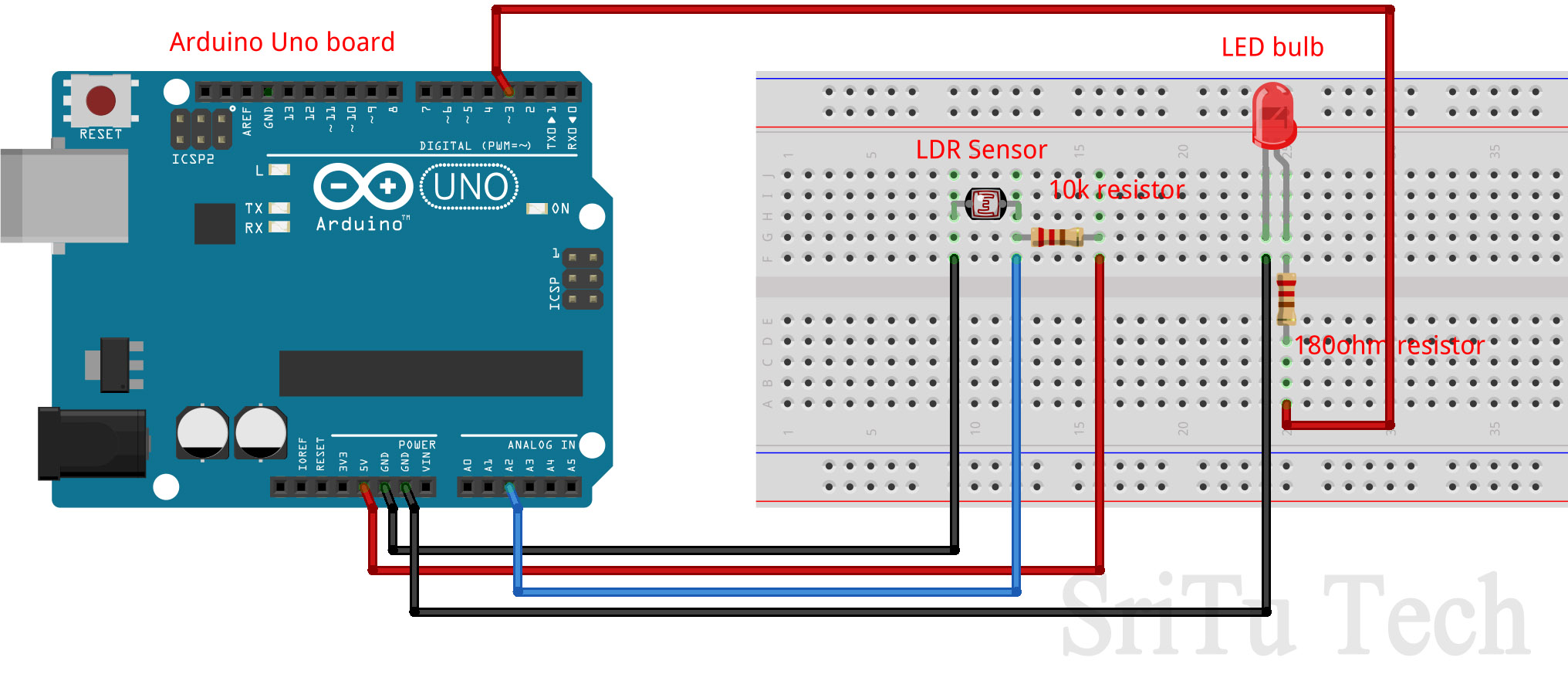### Step 3

Let’s look at the code below.
``````#define led 3
#define sensor A2

void setup() {
pinMode(led, OUTPUT);
Serial.begin(9600);
}
void loop() {
value = map(value, 0, 1023, 0, 255);
Serial.println(value);
analogWrite(led, value);
}``````
For the first time, we have defined the pins we use.
#define led 3
#define sensor A2
Then, the pin connected to the LED bulb is set to the output pin and the serial monitor is activated. These codes are included in the void setup function.
void setup() {
pinMode(led, OUTPUT);
Serial.begin(9600);
}

After that, the sensor value is read and put into the integer variable. The variable name is called “value”.

This code converts the value from 0 – 1024 to 0 – 255. This is because the PWM value ranges from 0 – 255.

value = map(value, 0, 1023, 0, 255);

Then, using this value, the LED bulb is turned on and off.

analogWrite(led, value);

This code With this code, the amount of light in the bulb increases or decreases depending on the amount of light received by the sensor. Look at the code below. This code turns on the LED bulb when the sensor value reaches a certain value or higher. For that, the if condition is used. Please upload and check these two codes separately.

``````/*LDR sensor control circuit.
created by the SriTu Tech team.
Read the code below and use it for any of your creation
*/
#define led 3 //led pin
#define sensor A2 //sensor pin

void setup() {
Serial.begin(9600);//serial monitor
pinMode(led, OUTPUT);
}

void loop() {
Serial.println(value);
if (value >= 950) {
digitalWrite(led, HIGH);//LED ON
Serial.println("LED ON");
} else {
digitalWrite(led, LOW);//LED OFF
Serial.println("LED Off");
}
}``````

OK Let’s upload the first code.

### Step 4

Select board and port.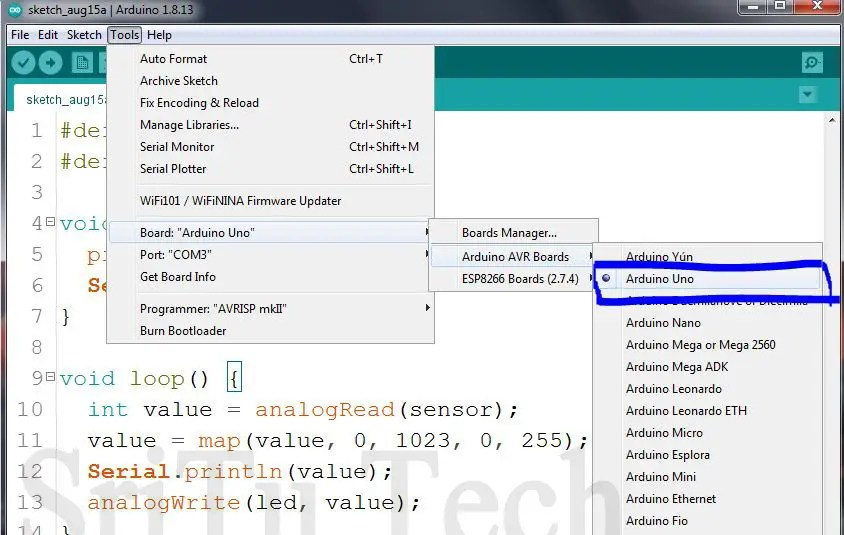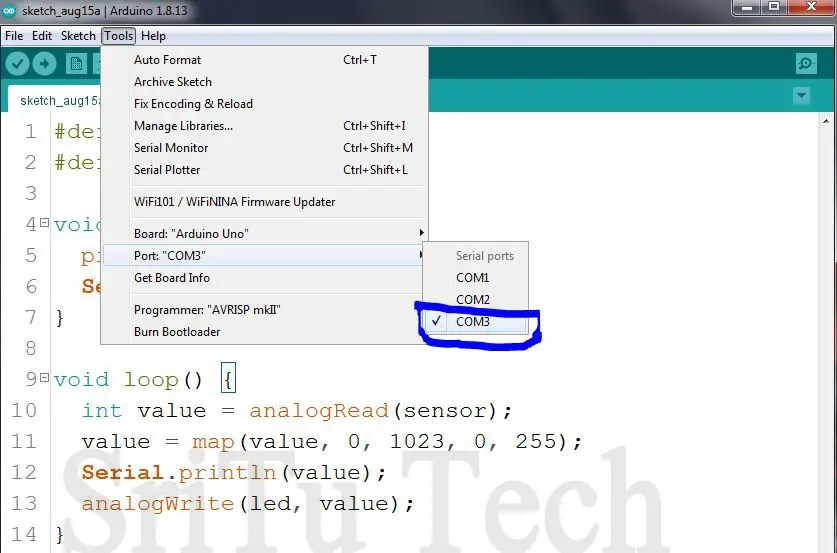### Step 5

Finally, upload the code and enjoy it.Everything is done. Test this circuit by increasing or decreasing the light. Please upload the second code and check it out. Below is a video of it being done. Please watch it. So, we will meet in the next post. Have a good day. Bye-bye.

LDR sensor control with Arduino

Shopping Cart
Scroll to Top